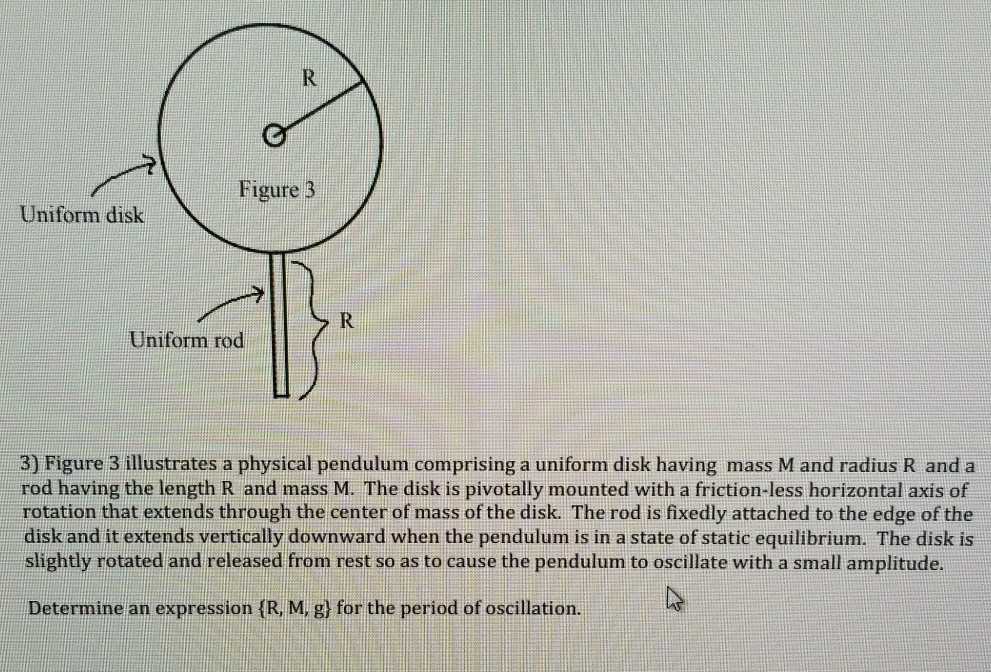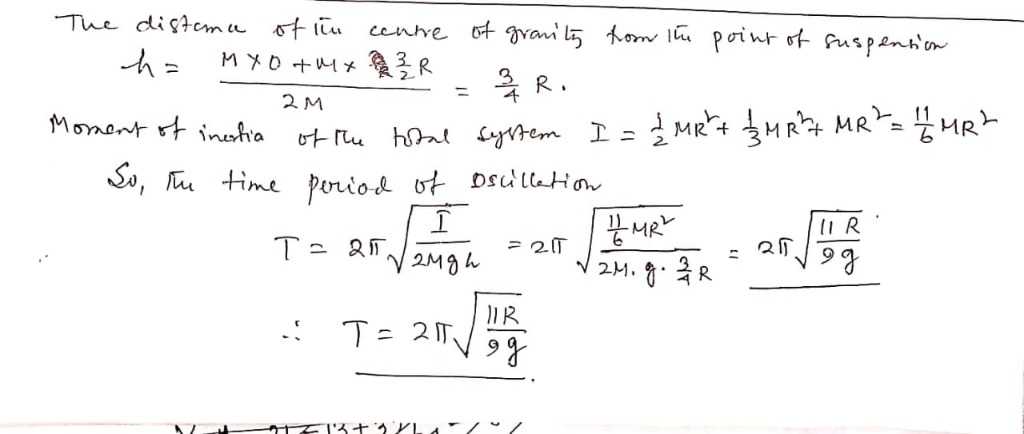# Figure 3 Uniform disk Uniform rod 3) Figure 3 illustrates a physical pendulum comprising a uniform disk having mass M and radius R and a rod having the length R and mass M. The disk is pivotally m...Figure 3 Uniform disk Uniform rod 3) Figure 3 illustrates a physical pendulum comprising a uniform disk having mass M and radius R and a rod having the length R and mass M. The disk is pivotally mounted with a friction-less horizontal axis of rotation that extends through the center of mass of the disk. The rod is fixedly attached to the edge of the disk and it extends vertically downward when the pendulum is in a state of static equilibrium. The disk is slightly rotated and released from rest so as to cause the pendulum to oscillate with a small amplitude. Determine an expression (R, Mi gl for the period of oscillation.##### Add Answer of: Figure 3 Uniform disk Uniform rod 3) Figure 3 illustrates a physical pendulum comprising a uniform disk having mass M and radius R and a rod having the length R and mass M. The disk is pivotally m...
More Homework Help Questions Additional questions in this topic.